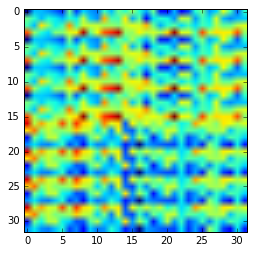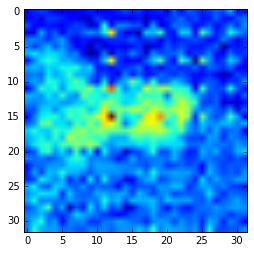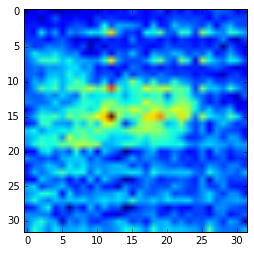# Calibrating images from Heimann thermopile arrays

After reading an image off a Heimann thermopile array, the pixel values can be converted to temperature readings through the use of calibration parameters stored on the device. To extract the calibration parameters, it is easiest to first read off the entire EEPROM on the thermopile array, as the Python script below does.

``````from periphery import I2C
import pickle

i2c = I2C("/dev/i2c-1")
query = [I2C.Message([0x00, 0x00]), I2C.Message([0x00]*8000, read=True)]
pickle.dump(query.data, open("eeprom.p", "wb"))
``````

Then, parameters and calibration values can be extracted from this array, as described in the Heimann datasheet.

``````VddComp = eeprom[0x0540:0x0740:2] + (eeprom[0x0541:0x0740:2] << 8)

ThGrad = eeprom[0x0740:0x0F40:2] + (eeprom[0x0741:0x0F40:2] << 8)
ThGrad = [tg - 65536 if tg >= 32768 else tg for tg in ThGrad]

ThOffset = eeprom[0x0F40:0x1740:2] + (eeprom[0x0F41:0x1740:2] << 8)
ThOffset = np.reshape(ThOffset, (32, 32))
ThOffset[16:,:] = np.flipud(ThOffset[16:,:])

P = eeprom[0x1740::2] + (eeprom[0x1741::2] << 8)
P = np.reshape(P, (32, 32))
P[16:, :] = np.flipud(P[16:,:])

epsilon = float(eeprom[0x000D])
GlobalGain = eeprom[0x0055] + (eeprom[0x0056] << 8)
Pmin = eeprom[0x0000:0x0004]
Pmax = eeprom[0x0004:0x0008]
Pmin = struct.unpack('f', reduce(lambda a,b: a+b, [chr(p) for p in Pmin]))
Pmax = struct.unpack('f', reduce(lambda a,b: a+b, [chr(p) for p in Pmax]))
PixC = (P * (Pmax - Pmin) / 65535. + Pmin) * (epsilon / 100) * float(GlobalGain) / 100

VddCalib = eeprom[0x0046] + (eeprom[0x0047] << 8)
Vdd = 3280.0
VddScaling = eeprom[0x004E]

PTAToffset = eeprom[0x0038:0x003c]
PTAToffset = struct.unpack('f', reduce(lambda a,b: a+b, [chr(p) for p in PTAToffset]))
``````

However, while using these parameters to correct the raw data from the sensor results in a better image, there is still significant fixed pattern noise. I think that I must be doing something incorrectly, or have a misunderstanding about the expected result.

The raw sensor data appears as follows – noisy, and dominated by the ADC readout block noise.Temperature compensation can be performed by using the `ThGrad` and `ThOffset` calibration parameters.

``````def t_comp(a):
comp = np.zeros((32,32))

for i in range(8):
# calculate ambient temperature
Ta = np.mean(a[i]) * PTATgradient + PTAToffset

# temperature compensated voltage

Vcomp = np.reshape(a,(32, 32)) - comp

return Vcomp
``````

This is clearly very important, as it results in a significantly improved image. You can now see the rough outline of a warm object (my face).However, the next step, which compensates for the supply voltage, does not further improve the image. In fact, it worsens the fixed pattern noise.

``````def vdd_comp(Vdd, Vcomp):
VVddComp = np.zeros((32,32))
for i in range(16):
for j in range(32):
VVddComp[i,j] = Vcomp[i,j] - (VddComp[(j+i*32) % 128] * (Vdd - float(VddCalib)/10))/pow(2, VddScaling)

for i in range(16,32):
for j in range(32):
VVddComp[i,j] = Vcomp[i,j] - (VddComp[((j+i*32) % 128) + 128] * (Vdd - float(VddCalib)/10))/pow(2, VddScaling)

return VVddComp
``````I am not sure why this is, or what can be done to further clean up these images.

The sensor also has the capability to read out voltages “blind,” capturing just the electrical offsets. Subtracting these offsets from the raw sensor data produces a usable image, without fixed pattern noise, though it does have a significant random noise component, as expected from a difference image. Due to this noise, and quantization noise, I do not believe that this method would be usable for imaging through a MWIR filter.